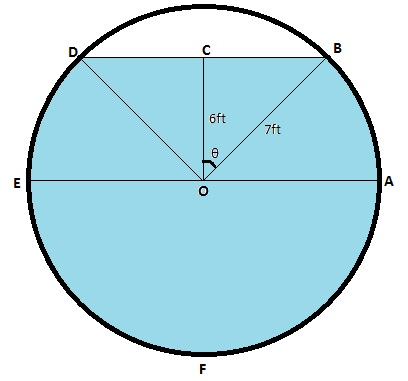# A water storage tank has the shape of a cylinder with diameter 14 ft. It is mounted so that the...

## Question:

A water storage tank has the shape of a cylinder with diameter 14 ft. It is mounted so that the circular cross-sections are vertical. If the depth of the water is 13 ft, what percentage of the total capacity is being used?

## Calculating Areas:

When dealing with geometrical shapes like cylindrical cross-sections, it helps to draw a figure based on the given information. Then geometrical results about area of a triangle and area of a sector of a circle can be used to calculate required areas.

## Answer and Explanation:

Answer: {eq}74.486\% {/eq}

Explanation:{eq}\mathrm{Percentage\, of\, total \,capacity\, used }\,=\, \displaystyle \frac {\mathrm{Area\,...

See full answer below.

Become a Study.com member to unlock this answer! Create your account

#### Learn more about this topic:

Finding the Area of a Sector: Formula & Practice Problems

from NY Regents Exam - Geometry: Help and Review

Chapter 6 / Lesson 14
55K